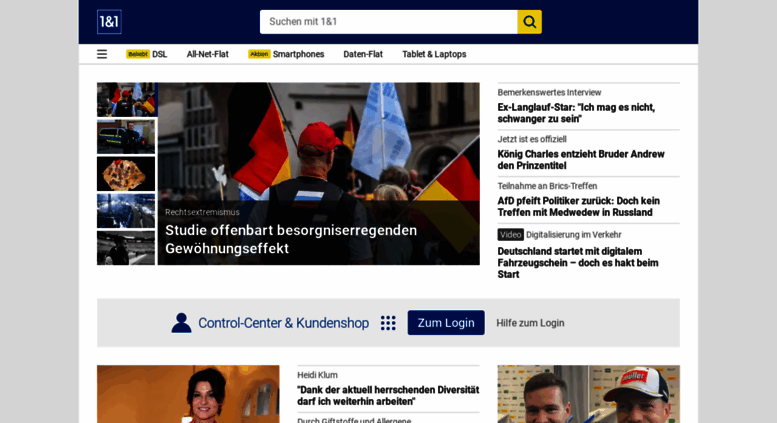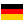# home.1und1.de: 1&1 - Telefon-Internet-Flatrates und mobiles Internet

Günstige Telefon-Internet-Flatrates: telefonieren und surfen zum kleinen Preis. Mit der Handy-Flatrate unbegrenzt in alle Netze telefonieren und mobil surfen!

• 59/100

Normal result
• 6

Successful tests
• 2

Failed tests
• First response

692 ms

3.3 sec

• Page rendered

600 ms

4.6 sec

Visit home.1und1.de now to see the best up-to-date Home 1 Und content for Germany and also check out these interesting facts you probably never knew about home.1und1.de

We analyzed Home.1und1.de page load time and found that the first response time was 692 ms and then it took 3.9 sec to load all DOM resources and completely render a web page. This is a poor result, as 60% of websites can load faster.

## Page optimization

•  HTML 241.0 kB Images 1.4 MB Javascripts 819.8 kB CSS 281.6 kB

In fact, the total size of Home.1und1.de main page is 2.7 MB. This result falls beyond the top 1M of websites and identifies a large and not optimized web page that may take ages to load. 70% of websites need less resources to load. Images take 1.4 MB which makes up the majority of the site volume.

• Original 241.0 kB
• After minification 182.4 kB
• After compression 34.1 kB

HTML optimization

HTML content can be minified and compressed by a website’s server. The most efficient way is to compress content using GZIP which reduces data amount travelling through the network between server and browser. This page needs HTML code to be minified as it can gain 58.6 kB, which is 24% of the original size. It is highly recommended that content of this web page should be compressed using GZIP, as it can save up to 206.9 kB or 86% of the original size.

• Original 1.4 MB
• After optimization 1.3 MB

Image optimization

Image size optimization can help to speed up a website loading time. The chart above shows the difference between the size before and after optimization. Home 1 Und images are well optimized though.

• Original 819.8 kB
• After minification 733.9 kB
• After compression 194.7 kB

JavaScript optimization

It’s better to minify JavaScript in order to improve website performance. The diagram shows the current total size of all JavaScript files against the prospective JavaScript size after its minification and compression. It is highly recommended that all JavaScript files should be compressed and minified as it can save up to 625.1 kB or 76% of the original size.

• Original 281.6 kB
• After minification 254.7 kB
• After compression 39.9 kB

CSS optimization

CSS files minification is very important to reduce a web page rendering time. The faster CSS files can load, the earlier a page can be rendered. Home.1und1.de needs all CSS files to be minified and compressed as it can save up to 241.7 kB or 86% of the original size.

### Network requests diagram

• home.1und1.de
• homepage.css
• homepage.js
• ngvar.js
• 31383756,pd=3,h=250,mxh=600,mxw=800,w=300.jpg
• 31383734,pd=4,h=250,mxh=600,mxw=800,w=300.jpg
• 31383124,pd=2,h=192,mxh=600,mxw=800,w=300.jpg
• 31383552,pd=3,h=250,mxh=600,mxw=800,w=300.jpg
• 31383600,pd=3,h=192,mxh=600,mxw=800,w=300.jpg
• 31383498,pd=6,h=192,mxh=600,mxw=800,w=300.jpg
• 31383600,pd=3,h=54,mxh=600,mxw=800,w=72.jpg
• 31383498,pd=6,h=54,mxh=600,mxw=800,w=72.jpg
• 31381734,pd=5,h=70,mxh=600,mxw=800,w=125.jpg
• 31383570,pd=3,h=250,mxh=600,mxw=800,w=300.jpg
• 31383408,pd=3,h=70,mxh=600,mxw=800,w=125.jpg
• 31381530,pd=2,h=192,mxh=600,mxw=800,w=300.jpg
• 31381530,pd=2,h=54,mxh=600,mxw=800,w=72.jpg
• 31383774,pd=3,h=250,mxh=600,mxw=800,w=300.jpg
• 31383600,pd=3,h=70,mxh=600,mxw=800,w=125.jpg
• 31382736,pd=3,h=70,mxh=600,mxw=800,w=125.jpg
• 31381506,pd=3,h=70,mxh=600,mxw=800,w=125.jpg
• 31375362,pd=1,h=192,mxh=600,mxw=800,w=300.jpg
• 31371756,pd=1,h=192,mxh=600,mxw=800,w=300.jpg
• 31375362,pd=1,h=54,mxh=600,mxw=800,w=72.jpg
• 31371756,pd=1,h=54,mxh=600,mxw=800,w=72.jpg
• 31382784,pd=2,h=250,mxh=600,mxw=800,w=300.jpg
• 31361496,pd=4,h=70,mxh=600,mxw=800,w=125.jpg
• 31381530,pd=2,h=250,mxh=600,mxw=800,w=300.jpg
• 30018444,pd=2,h=192,mxh=600,mxw=800,w=300.jpg
• 30018426,pd=2,h=192,mxh=600,mxw=800,w=300.jpg
• 30018432,pd=2,h=192,mxh=600,mxw=800,w=300.jpg
• 30018438,pd=2,h=192,mxh=600,mxw=800,w=300.jpg
• 30018444,pd=2,h=54,mxh=600,mxw=800,w=72.jpg
• 30018426,pd=2,h=54,mxh=600,mxw=800,w=72.jpg
• 30018432,pd=2,h=54,mxh=600,mxw=800,w=72.jpg
• 30018438,pd=2,h=54,mxh=600,mxw=800,w=72.jpg
• 31347444,pd=4,h=192,mxh=600,mxw=800,w=300.jpg
• 31358652,pd=3,h=192,mxh=600,mxw=800,w=300.jpg
• 31347444,pd=4,h=54,mxh=600,mxw=800,w=72.jpg
• 31358652,pd=3,h=54,mxh=600,mxw=800,w=72.jpg
• 31368294,pd=6,h=192,mxh=600,mxw=800,w=300.jpg
• 31368294,pd=6,h=54,mxh=600,mxw=800,w=72.jpg
• 31383584,pd=2,h=192,mxh=600,mxw=800,w=300.jpg
• 31383584,pd=2,h=54,mxh=600,mxw=800,w=72.jpg
• 31383638,pd=3,h=250,mxh=600,mxw=800,w=300.jpg
• 31383482,pd=5,h=70,mxh=600,mxw=800,w=125.jpg
• 31382798,pd=3,h=250,mxh=600,mxw=800,w=300.jpg
• 31369862,pd=5,h=192,mxh=600,mxw=800,w=300.jpg
• 31376852,pd=4,h=192,mxh=600,mxw=800,w=300.jpg
• 31369862,pd=5,h=54,mxh=600,mxw=800,w=72.jpg
• 31376852,pd=4,h=54,mxh=600,mxw=800,w=72.jpg
• 31382876,pd=3,h=70,mxh=600,mxw=800,w=125.jpg
• 31377032,pd=1,h=192,mxh=600,mxw=800,w=300.jpg
• 31377032,pd=1,h=54,mxh=600,mxw=800,w=72.jpg
• 31379378,pd=2,h=70,mxh=600,mxw=800,w=125.jpg
• 31376804,pd=3,h=70,mxh=600,mxw=800,w=125.jpg
• 31380548,pd=3,h=70,mxh=600,mxw=800,w=125.jpg
• 31363772,pd=3,h=70,mxh=600,mxw=800,w=125.jpg
• 31377848,pd=3,h=70,mxh=600,mxw=800,w=125.jpg
• 31146302,pd=5,h=250,mxh=600,mxw=800,w=300.jpg
• 31029956,pd=2,h=70,mxh=600,mxw=800,w=125.png
• 30918944,pd=2,h=70,mxh=600,mxw=800,w=125.jpg
• 30611246,pd=3,h=70,mxh=600,mxw=800,w=125.jpg
• 31344716,pd=2,h=192,mxh=600,mxw=800,w=300.jpg
• 31299890,pd=2,h=192,mxh=600,mxw=800,w=300.jpg
• 31344716,pd=2,h=54,mxh=600,mxw=800,w=72.jpg
• 31299890,pd=2,h=54,mxh=600,mxw=800,w=72.jpg
• 31341578,pd=3,h=192,mxh=600,mxw=800,w=300.jpg
• 31332428,pd=4,h=192,mxh=600,mxw=800,w=300.jpg
• 31341578,pd=3,h=54,mxh=600,mxw=800,w=72.jpg
• 31332428,pd=4,h=54,mxh=600,mxw=800,w=72.jpg
• 31341014,pd=3,h=192,mxh=600,mxw=800,w=300.jpg
• 31365686,pd=4,h=192,mxh=600,mxw=800,w=300.jpg
• 31341014,pd=3,h=54,mxh=600,mxw=800,w=72.jpg
• 31365686,pd=4,h=54,mxh=600,mxw=800,w=72.jpg
• 31367486,pd=2,h=192,mxh=600,mxw=800,w=300.jpg
• 31361456,pd=2,h=192,mxh=600,mxw=800,w=300.jpg
• chrome.png
• ff.png
• ie_img.png
• ie_home.png
• ico-startseite.png
• lotto_button.png
• eurojackpot_button.png
• emig.png
• 1und1_unterstuetzt_unicef.png
• footer-de-mail.png
• top_arbeitgeber_2016.png
• member-of-ui.png
• 31383124,pd=2,h=54,mxh=600,mxw=800,w=72.jpg
• 31382734,pd=3,h=250,mxh=600,mxw=800,w=300.jpg
• 31376014,pd=2,h=70,mxh=600,mxw=800,w=125.jpg
• 31382776,pd=2,h=192,mxh=600,mxw=800,w=300.jpg
• 31382776,pd=2,h=54,mxh=600,mxw=800,w=72.jpg
• 31382716,pd=2,h=250,mxh=600,mxw=800,w=300.jpg
• 31383124,pd=2,h=250,mxh=600,mxw=800,w=300.jpg
• 31379182,pd=4,h=250,mxh=600,mxw=800,w=300.jpg
• 31375666,pd=1,h=192,mxh=600,mxw=800,w=300.jpg
• 31375666,pd=1,h=54,mxh=600,mxw=800,w=72.jpg
• 30967936,pd=2,h=70,mxh=600,mxw=800,w=125.jpg
• 31375762,pd=4,h=192,mxh=600,mxw=800,w=300.jpg
• 31327546,pd=5,h=192,mxh=600,mxw=800,w=300.jpg
• 31375762,pd=4,h=54,mxh=600,mxw=800,w=72.jpg
• 31327546,pd=5,h=54,mxh=600,mxw=800,w=72.jpg
• 31333672,pd=6,h=192,mxh=600,mxw=800,w=300.jpg
• 31333672,pd=6,h=54,mxh=600,mxw=800,w=72.jpg
• 31359472,pd=2,h=192,mxh=600,mxw=800,w=300.jpg
• 31359472,pd=2,h=54,mxh=600,mxw=800,w=72.jpg
• logo.png
• sprite-weather.png
• icon-phone.png
• sticky-tabs.png
• search.png
• spinner.gif
• banner
• 31367486,pd=2,h=54,mxh=600,mxw=800,w=72.jpg
• 31361456,pd=2,h=54,mxh=600,mxw=800,w=72.jpg
• 31382808,pd=5,h=250,mxh=600,mxw=800,w=620.jpg
• 31371006,pd=2,h=192,mxh=600,mxw=800,w=300.jpg
• 31371006,pd=2,h=54,mxh=600,mxw=800,w=72.jpg
• content
• bounce
• PBX_ANF-NK_Feb2_g.jpg
• domain_box_l.jpg
• PBX_DSL_NK_Feb_g.jpg
• PBX_ANF-NK_Feb2_k.jpg
• domain_box_s.jpg
• PBX_DSL_BK_Feb_k.jpg
• content
• 1und1_firefox_med.png
• more_bright.png
• playbutton.png
• more.png
• 30064798,pd=1.jpg
• 30015564,pd=2.jpg
• 30468486,pd=1.jpg
• 30015590,pd=2.jpg
• auto-service-big.png
• auto-service-small.png
• banner
• 30015582,pd=2.jpg
• horoscope.png
• lotto_bg.jpg
• lotto_logo-2.png
• mioeuro.png
• lottokugel-2.png
• eurojackpot_bg.jpg
• lotto.png
• topde.png
• autoservice.png
• 30015586,pd=2.jpg
• 30015574,pd=2.jpg
• 30018180,pd=1.gif
• 30018176,pd=1.gif
• 30018188,pd=1.gif
• 30187850,pd=1.png
• 30018172,pd=2.png
• 30018184,pd=1.gif
• banner
• wpth.js
• a-ssl.ligatus.com
• SL_Logo_k.jpg
• SL_LOGO_G.jpg
• BPM_DSL_BK_JAN_k.jpg
• BPM_DSL_BK_JAN_g.jpg
• BPM_TabletFlat_BK_JAN_k.jpg
• BPM_Tablet-Flat_BK_JAN_g.jpg
• kind.jpg
• goldkurier_geldboerse_140x115.jpg
• Friendscout_Mann_jung_140x115.jpg
• PV_Female_HS_schwarzestiefel.jpg
• Koffer_150304.jpg
• Hemdenschwarz.jpg
• LederEtuikleider.jpg
• targeting.html
• banner
• s
• 2690.js
• 1und1.hp.js
• ico-sprungmarke.png
• s
• ttj
• lidar.js
• ligao.js
• t.gif
• browser_FF_24x24.png
• secondary_s.png
• close_bg_black.png
• close_black.png
• s
• activeview
• lighbs.js
• tx.io
• p.gif
• s
• tx.io
• blank.gif
• s
• 1%261.de_ui-responsivedesign-1450084024.php
• sync
• t1-1456237006.php

Our browser made a total of 217 requests to load all elements on the main page. We found that 0% of them (1 request) were addressed to the original Home.1und1.de, 29% (63 requests) were made to Img.ui-portal.de and 20% (43 requests) were made to I0.1und1.de. The less responsive or slowest element that took the longest time to load (1.2 sec) relates to the external source Img.ui-portal.de.

Requests

The browser has sent 211 CSS, Javascripts, AJAX and image requests in order to completely render the main page of Home 1 Und. We recommend that multiple CSS and JavaScript files should be merged into one by each type, as it can help reduce assets requests from 17 to 1 for JavaScripts and from 7 to 1 for CSS and as a result speed up the page load time.

17

Javascripts

181

Images

7

CSS

6

AJAX

211

All

Possible request optimization

1

Javascripts

160

Images

1

CSS

6

AJAX

43

Optimized

168

All

Normal result

This IP address is dedicated to Home.1und1.de. This is the best domain hosting practice .

Normal result

IP Trace

home.1und1.de

82.165.230.16

DNS records

Type Host Target/ip TTL Other
A

home.g-ha-1und1.de

82.165.230.16 30
CNAME

home.1und1.de

home.g-ha-1und1.de 300
TXT

home.g-ha-1und1.de

Language and encoding

Good result

Language

DEDetected

DEClaimed

Encoding

UTF-8

Claimed

Language claimed in HTML meta tag should match the language actually used on the web page. Otherwise Home.1und1.de can be misinterpreted by Google and other search engines. Our service has detected that German is used on the page, and it matches the claimed language. Our system also found out that Home.1und1.de main page’s claimed encoding is utf-8. Use of this encoding format is the best practice as the main page visitors from all over the world won’t have any issues with symbol transcription.

Similarly rated websites

HTTPS certificate

SSL Certificate

Home.1und1.de has no SSL certificate. Web browsing can be safer with HTTPS connection, so we suggest that it should be obtained for this site.

Poor result

Visitor World Map

Country of origin for 94% of all visits is Germany. It’s good for Home.1und1.de that their server is also located in Germany, as that enables the majority of their visitors to benefit from a much faster page load time.

Good result

Ratings of similarly popular websites

Poor result

Social Sharing Optimization

Open Graph description is not detected on the main page of Home 1 Und. Lack of Open Graph description can be counter-productive for their social media presence, as such a description allows converting a website homepage (or other pages) into good-looking, rich and well-structured posts, when it is being shared on Facebook and other social media. For example, adding the following code snippet into HTML <head> tag will help to represent this web page correctly in social networks:

home.1und1.de